## S-Domain Bode/Nyquist Plot

The Bode/Nyquist Plot applet now has a tab for impulse and step function responses. The previous version is still available here if you need it. As in the previous versions the focus remains on design of cascaded filter sections, so numerator and denominator are still entered as one or more polynomials, which get multiplied and terms collected before plotting. For discrete time investigations, try my new Z-Domain Bode/Nyquist Plot Java applet.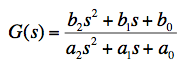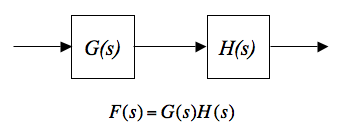In general, the transfer function of any linear system can be expressed as a ratio of two polynomials in powers of the Laplace variable s. The order of the transfer function is just the difference between the highest and lowest powers of s present. The general form shown above has powers ranging from zero to two, so the form shown is 2nd order. When two filter sections are cascaded, as with G(s) and H(s) above, the overall transfer function F(s) is the complex product of the two individual transfer functions. The steady-state gain for a continuous wave (CW) input is the absolute value of the transfer function, evaluated by setting s = where j is the square root of -1 and ω is the angular frequency of the input in units of radians/second. The phase shift for the same CW input is the argument (arctangent) of the transfer function at that frequency. The Bode Diagram gives a log-log plot of gain and a lin-log plot of phase, so the product of two transfer functions can be visualized by simply summing up the values. Following are some details of how to use the Bode/Nyquist Java Applet.

• The "Plot Response" button causes the plot to be recalculated and displayed whenever you click on it. Bode and Nyquist plots use the same data, and both are updated when you click this button. If the calculation fails due to bad inputs, a message will appear in the text area on the right.
• The "Hide Phase" check box does not cause a recalculation, but does redraw the window without phase information for the Bode Plot, and without negative frequency and unit circle for the Nyquist Plot. Similarly, the step function response is hidden on the Impulse tab.
• The "Decades" combo box lets you select 2, 3, or 4 decades of frequency in the horizontal axis. The same frequency range will be used for the Nyquist Plot. This control always causes the plot to be recalculated and redrawn.
• The "Units" combo box lets you select units of "Hz" or "rad/sec" for the Bode Plot. Internal calculations are always done in radians/second, but the horizontal axis labels will follow this control, as will the frequency values given in the right-hand text area.
• The "Numerator" field is where you type transfer function coefficients for the polynomial in the numerator, representing a sum in powers of the Laplace variable s. To simplify parsing this field, you just enter the coefficients in order from highest order to lowest, always ending with the zeroth order coefficient on the right. Leading zeros are optional, trailing zeros aren't. Coefficients are separated with commas or spaces, noting that consecutive commas or spaces count as one.
• Similarly, the "Denominator" field is where you type transfer function coefficients for the polynomial in the denominator. If several polynomials are to be multiplied to form the numerator or denominator, enclose each set of coefficients within parentheses. As a shortcut you can use a semicolon ';' to separate polynomials instead of parentheses. Operation of nested parentheses is not defined. All coefficients are real numbers which may be entered as decimal values (1.234) or using scientific notation (1.23e4).
• The "Start Freq." field lets you specify the frequency in the selected units at the left edge of the Bode Plot. All frequencies indicated on the plot are then calculated from the start frequency. For instance, if you want to plot two decades with 1 rad/sec in the center, start the plot at 0.1 rad/sec. Or to plot the audio range from 20 Hz to 20 kHz, plot three decades starting at 20 Hz. Any real number can be entered, either as a decimal value or using scientific notation. Negative numbers are allowed, and are interpreted as a 180° phase shift. The start frequency is also used to scale the horizontal axis for impulse and step function responses.
• The text area on the right contains the complex value of the transfer function as it varies with frequency, listed in two columns, for the Bode plot and the Nyquist plot tabs. The first column is the real value of the frequency in the selected units using scientific notation. The second column is the complex value of the transfer function expressed in a format that can be pasted into Excel spreadsheets when the Analysis ToolPak is enabled. See the heading "Software Development Issues" below for more discussion. For the Impulse function tab, the text area contains a time series with time (units are seconds) in the first column, impulse function response in second column, and step function response in the third column.

### Software Verification

While developing the applet I kept in mind this short list of simple test cases with known results, which allowed me to quickly verify that the applet actually works. Type the numerator and denominator strings into the applet's entry fields and click "Plot Response" to see the result. Corner or center frequency ω0 in each case is 1 radian/second, or about 0.1592 Hz. These sections can be cascaded in combination, and scaled in amplitude or frequency for further tests. Diagnostic information is sent to the Java Console to help you better understand the applet's operation.

Table 1. Verification Test Cases
DescriptionNumeratorDenominatorNotes
Integrator(1)(1,0)Unity gain at ω0, constant phase of -90°
Differentiator(1,0)(1)Unity gain at ω0, constant phase of +90°
2nd order low pass(1)(1,1.414,1)-3 dB gain at ω0, phase crosses -90° at ω0
Bandpass filter(1,0)(1,.25,1)+12 dB gain at ω0, phase crosses 0° at ω0
2nd order high pass(1,0,0)(1,1.414,1)-3 dB gain at ω0, phase crosses +90° at ω0
Notch filter(1,0,1)(1,1,1)Notch centered at ω0, phase flips from -90 to +90° at ω0
2nd order phase shift(1,-1,1)(1,1,1)Unity gain at all frequencies, phase crosses 180° at ω0

### Software Development Issues

• Data transfer through the system clipboard has been a source of trouble since this has been identified as a potential security risk, where the bad guy hopes you'll leave a password or other sensitive information on the clipboard where he can find it later. And now Java itself has become known as a security risk, leading to ever-tightening restrictions on its use in web browsers. If you can see the applets on this page, it's likely that you've already jumped through a number of hoops to get through the security restrictions. I've looked into buying a signing certificate for my jar files, but found that it's difficult, expensive, and meant for businesses, not individual authors. If you would like to use my applets without restriction, especially the copy-and-paste features, then you should consider downloading my jar file and running it locally, as described below. Or you can download my source file (link given below) and build the applet yourself. The Eclipse IDE is especially convenient for this.
• I've chosen to give the complex response of the transfer function in the right-hand text area in a format which I've found works in Excel when you enable Microsoft's Analysis ToolPak Add-In, required to work with complex numbers. This works reliably for me when pasting from Java into various flavors of Excel. Excel however has a quirk (defect appears in all versions, both Mac and PC, that I have access to) that complex values with a negative real part can't be entered manually because of the leading minus sign. Same defect observed entering complex values with a leading plus sign. Luckily I have not seen this defect when pasting from other applications like the Bode/Nyquist applet. A simple workaround is to place a single-quote sign "'" in front of the leading minus sign before hitting [ENTER]. This forces Excel to treat the complex number as a text string, which is what it does anyways unless it's actually doing a calculation.
• Drawing the Bode Plot is a fairly simple process, but there is one subtlety. We expect the plot to be smooth in some regions, and to have singularities in others, particularly where poles and zeroes are found. These features tend towards infinite slope which is hard to plot accurately. The design presented here is a brute-force solution which calculates the complex value of the transfer function at 600 distinct frequencies, one for each column of pixels in the plot window. One can imagine more sophisticated solutions that adapt to slope as it varies, but the brute-force approach allows us to show all features wider than one pixel regardless of slope. Modern computers can calculate all 600 points without breaking a sweat.
• The Impulse response tab, new in this version, plots an estimate of the response of your transfer function to an impulse function input, obtained by computing the inverse Fourier transform of the transfer function. The step function response is obtained by numerically integrating (summing up) the impulse function response. This technique is useful, but does leave artifacts in many cases. Also it tends to diverge for transfer functions with unbounded responses at high or low frequencies, including simple integrators and differentiators. In some cases you can reduce artifacts by increasing the start frequency, which is used to determine the details of the inverse Fourier transform. The input to the transform is the complex response of the transfer function at 512 frequencies, starting with -256 times the start frequency and ending with 255 times the start frequency. The output is a time series of 512 real-valued points comprising one period of the lowest frequency present in the input, which is the start frequency. Let's say you wanted to plot an impulse response at 44100 samples per second: 512 samples would take 11.61 msec which is one period of 86.1328 Hz or 541.188 radians/second. Setting the start frequency to these numbers will then give the desired plot. Note that the Fourier technique assumes that the time series is periodic, which means that if we were to plot more than 512 points we would see multiple copies of the impulse response. If you enter a negative start frequency, time (in the horizontal axis) runs backwards making the plot hard to interpret.
• You'll notice that I've placed multiple instances of the applet on this web page. Behind the scenes however, your browser is using the same jar file for all of them. Each instance runs independently so there is no conflict or confusion. In fact, you can open multiple windows or tabs containing this page to compare various filter tunings side by side. You can also run multiple instances of Bode/Nyquist Plot locally as Java applications. On PCs just create a desktop shortcut with a command line like "java -cp c:\somePath\BodeS.jar BodeSPackage.BodeS". A similar mechanism exists for Macintosh OS X, but with a few more steps.
• The complete source code and executable for this new version are available for free download here. Single-right-click (CTRL-click for Mac) on these links to save local copies: The jar file was compiled with JDK 1.5. The user interface is based on the Java Swing model at version 1.3, and should build and run without error on all newer versions. The Bode/Nyquist applet may be run in a browser over a network or locally, and may also be run as a standalone Java application without modification. Of course it runs in AppletViewer as well. Please remember that, as with previous versions, only you can determine if this applet is usable in your application, and only you know if you are qualified to make modifications. In order to run on as wide a variety of platforms and versions as possible, I've used applet tags in my HTML.
• I haven't implemented any code to help with printing the applet window from your browser. Some browsers print it just fine, others leave a blank hole where the applet should be. Of course, you can always use a screen grabber to capture and print the applet window.

### Hardware VerificationThe real utility of this applet isn't in matching well-known textbook cases, but in accurately predicting the performance of actual filters that you design and build. To that end, I've studied two simple design examples shown below, taken from the world of real-time audio analyzers (RTAs). Both examples use cascaded filter sections to build up complicated transfer functions. In each case, output from the Bode/Nyquist applet closely matches measured results obtained on my bench with a simple breadboard. Unless otherwise noted, all opamps are Texas Instruments TLC2272CP (operating with filtered, regulated +/- 5 Volt supplies), all resistors are 5% 1/4 Watt carbon film (in series or parallel combination of at most 2 resistors, to get close to desired value), and all capacitors are polypropylene film, Panasonic P-Series (2% tolerance at nominal value).

### Flat-Top Bandpass Filter Design Example

A real-time analyzer operates by separating its input into discrete channels and showing each channel's amplitude separately. An octave-band analyzer, for instance, might separate its input into ten octave-wide channels with center frequencies ranging from 32 Hz to 16 kHz. Here we consider the 1 kHz filter response which will be fourth order to reject adjacent channel signals, and will be peaked at two frequencies with an offset to flatten response within the passband. The slight dip at the center frequency is a design tradeoff that can be varied to meet your needs.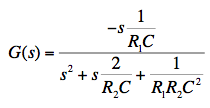As Don Lancaster describes in his "Active Filter Cookbook" (Lancaster ) the two-pole fourth-order bandpass response is well-suited for implementing a flat-top bandpass filter. Following his design process, we set the staggering factor a = 4/3, or about 1.333, and Q = 2.5. This will leave us with a -0.5 dB dip at the center frequency, which is quite reasonable for use in a real time analyzer. We'll cascade two sections of the type shown in Fig. 2 above, which is a single-amplifier multiple-feedback bandpass filter. Starting with all component values set to unity (1 Ω and 1 Farad), the center frequency will be 1 radian/second. First I'll change the capacitors to a more practical value of 0.01 μF, which causes a corresponding change in center frequency to 108 rad/sec., or about 15.92 MHz. Both capacitors maintain the same value in this design. Next I change both resistors to 15.92 kΩ to drop the center frequency back to 1 kHz. Then, following Lancaster's recipe, I decrease the input resistor R1 by a factor of 1/(2Q) to 3184 Ω, and increase the feedback resistor R2 by a factor of 2Q to 79.6 kΩ. Finally, I multiply both resistors by a to get the lower section values, and divide both resistors by a to get the upper section values. The results of all this figuring are shown in the table below.

Table 2. Component Values for 1 kHz Flat-Top Bandpass Filter
Lower Section (f0 = 750 Hz)Upper Section (f0 = 1333 Hz)
ResistorsR1 = 4245 Ω, R2 = 106.1 kΩR3 = 2388 Ω, R4 = 59.7 kΩ
CapacitorsC1 = C2 = 0.01 μFC3 = C4 = 0.01 μF
Numerators(-23560,0)(-41880,0)
Denominators(1,1885,22200000)(1,3350,70140000)

Plugging the parts values given above into the transfer function of Fig. 2, we obtain the transfer function coefficients, shown rounded to 4 significant figures in Table 2 above. Entering the numerator and denominator coefficients into the Bode/Nyquist applet and setting the start frequency to 10 Hz (already done for you in the HTML above), we find the desired flat-top peak response. In addition, we see that overall phase crosses zero at exactly 1 kHz, hits +90° at 707 Hz, and -90° at 1414 Hz. For use in a real-time analyzer one would want the peak gain to be unity, but here we actually have excess gain of about 34 dB over the two filter sections. A simple resistive attenuator (not shown here) in front of each section could return overall gain to unity. Note that the output impedance of the resistive attenuator would have to be accounted for as part of the input resistors R1 and R3.The order in which we cascade the sections has no effect on overall frequency response so we can arrange them to best suit our needs. In this case I've placed the lower section first because it has a higher input impedance. I added the trimmers R5 and R6 (each 1 kΩ, and I reduced R1 and R3 by 500 Ω each) to my breadboard so I could independently adjust the center frequency of each section while accounting for any source impedance seen at the inputs. Unless you are driving the filter with a stiff voltage source and buy precision components for each value, you'll want to align the filter to get the desired response. The procedure is quite easy due to the cascaded design. The input and output of each section can be directly probed to check relative phase shift across the section. Apply a continuous wave (CW) source to the overall input at the desired center frequency for each section one at a time, and adjust the appropriate input resistor (R5 or R6) to get exactly 180° of phase shift across the section you are aligning. You can watch the input and output in a Lissajous pattern on an X-Y oscilloscope, and trim the section's input resistor until the oval-shaped pattern closes to a straight diagonal line. Calibrate your scope probes if appropriate by connecting both X and Y probes to the same circuit node and verifying that the trace is a straight diagonal line. Once each of the sections is tuned to its desired center frequency, everything else will fall into place with measured response matching the calculated response within a fraction of a dB.

### Pinking Filter Design Example

Also from the realm of real-time-analyzers (RTAs), the pinking filter is used to turn white noise from a Gaussian source (equal energy per Hz) into pink noise (equal energy per octave) suitable for studying room acoustics in a real-time measurement. Assuming we have an ideal white noise source, perhaps a maximum-length-sequence (MLS) pseudo-random number generator, we will need a filter with a slope of exactly -10 dB/decade (approx. -3 dB/8va) over three decades of frequency from 20 Hz to 20 kHz. The problem is that traditional filter sections all come with slopes in multiples of 6 dB/8va. The technique I'll use here is to take a standard Sallen-Key (Sallen and Key ) low pass section with a slope of -12 dB/8va and relax its characteristics until the slope is just -3 dB/8va over about two decades of frequency.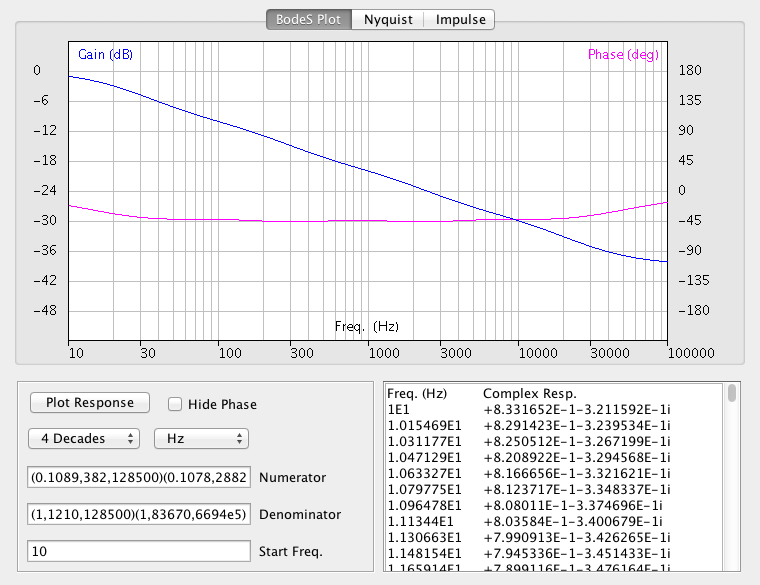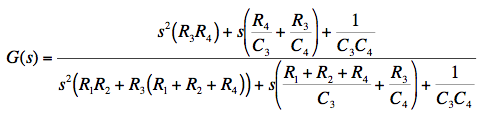This is done by shifting the capacitor values around, and adding resistors R3 and R4 in series with them. A second section of the same type is then added with its frequency range shifted up by two decades. After tweaking some component values, the smooth merged response shown above is obtained. Ideal component values, which reduce deviation to less than +/- 0.2 dB, are shown in Table 3 below. Plugging these ideal values into the transfer function of Fig. 4 gives the polynomial coefficients entered into the applet above, and also shown in Table 3. I found it convenient to keep the parts values in a spreadsheet which then calculates the coefficients.

Table 3. Ideal Component Values for Noise Pinking Filter
Lower Section (10 Hz...1 kHz)Upper Section (1 kHz...100 kHz)
ResistorsR1 = 10.02 kΩ, R2 = 9943 Ω
R3 = 2107 Ω, R4 = 8224 Ω
R5 = R6 = 11.91 kΩ
R7 = 2031 Ω, R8 = 11.31 kΩ
CapacitorsC3 = 0.1517 μF, C4 = 0.3226 μFC7 = 2.036 nF, C8 = 3.439 nF
Numerators(0.1089,382,128500)(0.1078,28820,6694e5)
Denominators(1,1210,128500)(1,83670,6694e5)
Deviation+/- 0.2 dB, 15 Hz...50 kHzI wanted to find out the error if I stuck to standard component values, so I tried several variations and wound up with the numbers in Table 4 below. I set five of the resistors to 10 kΩ so I could use a dual-inline-package (DIP) resistor network. Deviation is still within +/- 0.5 dB across the audio range, and then some. I was pleasantly surprised to find that the calculated and measured response for this alignment were indistinguishable (delta < 0.1 dB) in my test setup.

Table 4. Standard Component Values for Noise Pinking Filter
Lower Section (10 Hz...1 kHz)Upper Section (1 kHz...100 kHz)
ResistorsR1 = R2 = 10 kΩ
R3 = 2.2 kΩ, R4 = 6.8k Ω
R5 = R6 = R8 = 10 kΩ
R7 = 2.2 kΩ
CapacitorsC3 = 0.1 μF, C4 = 0.33 μFC7 = 2.2 nF, C8 = 3.3 nF
Numerators(1496e4,7467e7,3030e10)(2200e4,5212e9,1377e14)
Denominators(1590e5,2747e8,3030e10)(1660e5,1430e10,1377e14)
Deviation+/- 0.5 dB, 15 Hz...50 kHz

While gathering information for this write-up, I found several published schematics for passive pinking filters of similar complexity (say four resistors and four capacitors, GR 1390-P2) and tried to work out their transfer functions. Trouble was (and this is common to many passive filter designs) that all the parts interact so one winds up with a blizzard of terms that are very hard to put in standard form. I ultimately gave up on finding the transfer function and used a different technique to convince myself that while they do produce the desired -10 db/decade slope, passive pinking filters have more deviation over a smaller frequency range than the active design presented here.

### Resources on the Web

• The Bode/Nyquist Applet has been written to have the features I need in my work, but you may find that your needs differ. If so, you might consider alternatives like the PoleZero Applet from Brian Williams at MIT.
• Don't feel left out if you want to study Bode plots on your iPhone or iPad. See for instance the Bode & Nyquist iPhone App, from Laura Villani.
• CadSoft EAGLE design software was used to create the schematic diagrams shown above. Has lots of features and is fully cross-platform capable.
• Wikipedia maintains an article on the Colors of Noise, with samples of each type.
• Simply Noise has an Online Noise Generator that can be used for simple experiments with your sound card and computer speakers.
• Wikipedia gives lists of Preferred Values for Components which you may find helpful in designing filters.
• Hank Zumbahlen of Analog Devices has written a white paper on Phase Relations in Active Filters. In audio work we tend to emphasize amplitude response, but trust me, phase matters!

### References

• "Active Filter Cookbook" (2nd Ed.), Don Lancaster, Synergetics Press, Thatcher 1995.
• "A practical method of designing RC active filters", R.P. Sallen and E.L. Key, IRE Trans. Circuit Theory, vol. CT-2, pp. 74-85, March 1955. I put some effort into finding this paper, but it turns out to be pretty obscure, so I haven't read it. You may prefer to read about it at: Sallen-Key Topology, on Wikipedia, The Free Encyclopedia.
• "Just Java 2" (5th Ed.), Peter van der Linden, Sun Microsystems Press (Prentice Hall), Palo Alto 2002.
• "Feedback Control Systems" (3rd Ed.), C.L. Phillips & R.D. Harbor, Prentice Hall, Englewood Cliffs 1996.
• The General Radio 1390-P2 passive pink noise filter schematic is available in the GR 1390B User's Manual (see p.5).

### Fine Print

I am placing the ideas and source code presented on this page in the public domain, for the benefit of software developers and engineers. Please remember that only you can determine if these ideas are suitable for your application. The burden is on you to verify, through functional test, code inspection, and any other means you determine is appropriate, whether the source code presented here actually works or not. And only you know if you are qualified to make modifications to the source code presented here. Feel free to post a link to this page on your site, but please don't copy my content. This page should be available at this URL for the foreseeable future. I claim a trademark interest in the names "Williamsonic" and "www.williamsonic.com".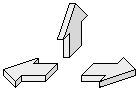Last updated 3/8/2015 by